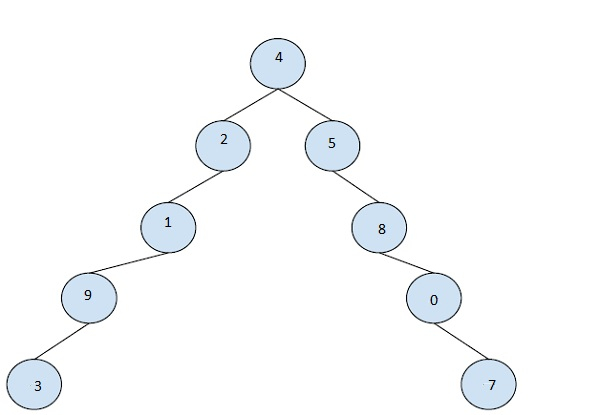# Find right sibling of a binary tree with parent pointers in C++

In this problem we are given a binary tree and parent pointers. Our task is to Find right sibling of a binary tree with parent pointers.

Let’s take an example to understand the problem,

## InputNode = 3

## Output

7

Solution Approach

A simple solution to the problem is finding the leaf node of the nearest ancestor (which is neither the current node nor the parest of the current node) which is at the same level as the current node. This is done by counting the levels while going up and then when coming down counting them down. And then finding the node.

Program to illustrate the working of our solution,

## Example

Live Demo

#include <bits/stdc++.h>
using namespace std;
struct Node {
int data;
Node *left, *right, *parent;
};
Node* newNode(int item, Node* parent) {
Node* temp = new Node;
temp->data = item;
temp->left = temp->right = NULL;
temp->parent = parent;
return temp;
}
Node* findRightSiblingNodeBT(Node* node, int level) {
if (node == NULL || node->parent == NULL)
return NULL;
while (node->parent->right == node ||
(node->parent->right == NULL && node->parent->left == node)) {
if (node->parent == NULL || node->parent->parent == NULL)
return NULL;
node = node->parent;
level++;
}
node = node->parent->right;
if (node == NULL)
return NULL;
while (level > 0) {
if (node->left != NULL)
node = node->left;
else if (node->right != NULL)
node = node->right;
else
break;
level--;
}
if (level == 0)
return node;
return findRightSiblingNodeBT(node, level);
}
int main(){
Node* root = newNode(4, NULL);
root->left = newNode(2, root);
root->right = newNode(5, root);
root->left->left = newNode(1, root->left);
root->left->left->left = newNode(9, root->left->left);
root->left->left->left->left = newNode(3, root->left->left->left);
root->right->right = newNode(8, root->right);
root->right->right->right = newNode(0, root->right->right);
root->right->right->right->right = newNode(7, root->right->right->right);
Node * currentNode = root->left->left->left->left;
cout<<"The current node is "<<currentNode->data<<endl;
Node* rightSibling = findRightSiblingNodeBT(currentNode, 0);
if (rightSibling)
cout<<"The right sibling of the current node is "<<rightSibling->data;
else
cout<<"No right siblings found!";
return 0;
}

## Output

The current node is 3
The right sibling of the current node is 7